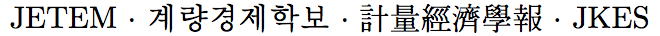Journal of Economic Theory and Econometrics: Journal of the Korean Econometric Society
Home

Archive

Search

Journal of Economic Theory and EconometricsJournal of the Korean Econometric Society

• #### Luc Rey-Bellet   (University of Massachusetts Amherst)Abstract

We provide several tests to determine whether a game is a potential game or whether it is a zero-sum equivalent game-a game which is strategically equivalent to a zero-sum game in the same way that a potential game is strategically equivalent to a common interest game. We present a unified framework applicable for both potential and zero-sum equivalent games by deriving a simple but useful characterization of these games. This allows us to re-derive known criteria for potential games, as well as obtain several new criteria. In particular, we prove (1) new integral tests for potential games and for zero-sum equivalent games, (2) a new derivative test for zero-sum equivalent games, and (3) a new representation characterization for zero-sum equivalent games.

Keywords
Potential Games, Zero-Sum Games, Zero-Sum Equivalent Games

JEL classification codes
C72, C73